# Integral equation with symmetric kernel

An integral equation with a real symmetric kernel (cf. Kernel of an integral operator):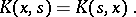The theory of linear equations with real symmetric kernel was first constructed by D. Hilbert (1904) by drawing upon the theory of symmetric quadratic forms and going over from a finite to an infinite number of variables. Shortly after this, E. Schmidt (1907) put forward a more elementary method of substantiating Hilbert's results. For this reason, the theory of integral equations with symmetric kernel is also called the Hilbert–Schmidt theory. A significant weakening of the restrictions imposed in this theory on the data and unknown elements was achieved by T. Carleman (see below).

Consider an integral equation of the second kind with real symmetric kernel:(1)

In the construction of the theory of integral equations with symmetric kernel, it suffices to suppose that the symmetric kernelis measurable on the square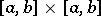and that(2)

while the free termand the unknown functionare square-integrable functions on(the integral being understood in the sense of Lebesgue).

The development of the theory of integral equations with symmetric kernel begins with the study of a number of general properties of the eigen values and functions of the homogeneous symmetric integral equation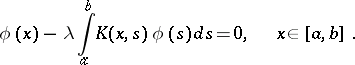(3)

Thus it is proved that: equation (3) has at least one eigen value (whenis almost-everywhere non-zero); eigen functions corresponding to distinct eigen values are orthogonal; the eigen values are real; since the kernel is real, it can be assumed without loss of generality that the eigen functions are real; and there are only a finite number of eigen values on any finite interval of values for the parameter.

The set of eigen values of (3) is called the spectrum of the equation. The spectrum is a non-empty finite or countable set of numbers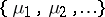; to eachof the spectrum corresponds a finite set of linearly independent eigen functions. The eigen values and eigen functions can be arranged as two sequences: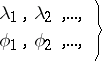(4)

such that the absolute values of the eigen values are non-decreasing: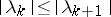, and each eigen value is repeated as many times as there are eigen functions corresponding to it. Thus, to each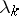in (4) corresponds just one eigen function. The system of eigen functions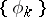can be assumed to be orthonormal. The sequence (4) is called a system of eigen values and eigen functions (or an eigen system) of the symmetric kernelor of the equation (3). The determination of this system is equivalent to the complete solution of the homogeneous symmetric integral equation (3).

The Fourier series of the kernel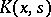, regarded as a function of, with respect to the orthonormal system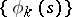is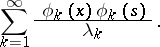(5)

This series formed from the eigen system of the symmetric kernelis called the bilinear series of, or the bilinear expansion ofin eigen functions. This series converges in the mean to, that is,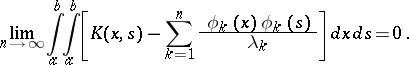If, in addition, the bilinear series (5) converges uniformly, then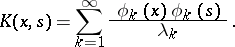In particular, the latter equality always holds if the kernel has only a finite number of eigen values. In this case the kernelis degenerate (cf. Degenerate kernel).

The converse statement also holds: A degenerate symmetric kernel has a finite number of eigen values (and, hence, a finite set of eigen functions). The bilinear series of a kernelthat is continuous on the squareand has positive eigen values converges uniformly.

Knowledge of the eigen system (4) enables one to construct the solution of the inhomogeneous equation (1). The following theorems hold.

Ifis not an eigen value of, then the symmetric integral equation (1) has a unique solution, given by the formula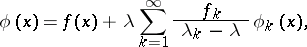(6)

whereare the eigen values and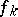are the Fourier coefficients ofwith respect to the orthogonal system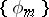of eigen functions of the kernel, in other words,Let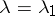be an eigen value of. Then the symmetric integral equation (1) is solvable if and only if the following conditions hold: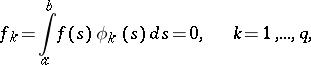where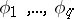are the eigen functions corresponding to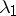. If these conditions are fulfilled, then all solutions of (1) are expressed by the formula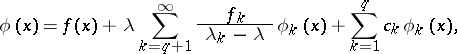(7)

where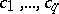are arbitrary constants.

Ifhas an infinite number of eigen values and, consequently, there are infinite series on the right-hand sides of the formulas (6), (7), then these series converge in the mean. If it is further required thatsatisfies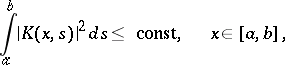then the above series converge absolutely and uniformly.

Formulas (6) and (7) are called Schmidt's formulas. Much of the theory of integral equations with symmetric kernel extends easily to complex-valued functions. In this case, the analogue of the real symmetric kernel is a Hermitian kernel: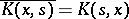.

If the eigen system (4) of a symmetric kernelis known, then it is easy to study the symmetric Fredholm equation of the first kind(8)

Suppose again that the symmetric kernelof equation (8) is square integrable on the squareand that the right-hand sideand the unknown functionare square-integrable functions on.

The symmetric kernel is called complete it its system of eigen functions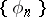is complete (closed) (cf. Complete system of functions).

Picard's theorem. Letbe a complete kernel. Then equation (8) is a solvable if and only if the seriesconverges, whereare the Fourier coefficients of. When the condition holds, the (unique) solution is expressible in the form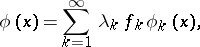(9)

and this series converges in the mean.

Carleman  has a constructed a theory under less restrictive conditions on the symmetric kernelthan those of Hilbert and Schmidt. These conditions are as follows: 1)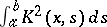exists in the sense of Lebesgue andfor any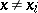, where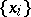is some sequence of points which may have a finite number of limit points; 2) there may exist a finite set of points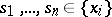in a neighbourhood of which the function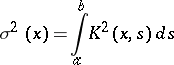is not integrable, but it must be integrable on the set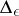obtained by removing from the intervalthe intervals,, whereis an arbitrarily small positive number.

Let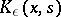be the function that vanishes on the set of points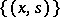for which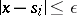,,, and that is equal to the kernelof equation (1) on the set of points ofoutside this set. The idea of Carleman's method is as follows:

Instead of equation (1) one considers the linear integral equation of the second kind with kernel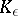. One studies the spectrum and solutions of this equation and then one investigates the spectrum and solution of equation (1) by passing to the limit as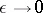.